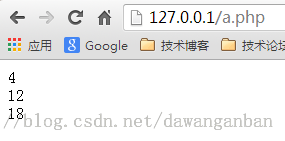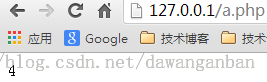淄博建站哪家好-九科数据网站建设！我们是一家专注于大中小型企业网站制作、营销型、响应式建站的淄博网络公司！PHP移动互联网开发笔记（4）——自定义函数及数

30Aug
2017

function funname(arg1, arg2, arg3......){

//TODO

return values;

}

1. 	<?php
function fun(\$m, \$n){
if(\$m==0 || \$n==0){
return 0;
}else{
\$a=\$m*\$n;
return \$a;
}
}

\$p=2;
\$h=3;
echo \$p."*".\$h."=".fun(\$p,\$h);
?><?php
/*
function fun(\$m, \$n){
if(\$m==0 || \$n==0){
return 0;
}else{
\$a=\$m*\$n;
return \$a;
}
}

\$p=2;
\$h=3;
echo \$p."*".\$h."=".fun(\$p,\$h); */

function fun(\$m, \$n=1, \$x=2){
\$a=\$m*\$n*\$x;
return \$a;
}

\$p=2;
echo fun(\$p)."<br>";          // 2*1*2 = 4
echo fun(\$p, 3)."<br>";       // 2*3*2 = 12
echo fun(\$p, 3, 3)."<br>";    // 2*3*3 = 18
?><?php
/*
function fun(\$m, \$n){
if(\$m==0 || \$n==0){
return 0;
}else{
\$a=\$m*\$n;
return \$a;
}
}

\$p=2;
\$h=3;
echo \$p."*".\$h."=".fun(\$p,\$h);
*/

/*
function fun(\$m, \$n=1, \$x=2){
\$a=\$m*\$n*\$x;
return \$a;
}

\$p=2;
echo fun(\$p)."<br>";          // 2*1*2 = 4
echo fun(\$p, 3)."<br>";       // 2*3*2 = 12
echo fun(\$p, 3, 3)."<br>";    // 2*3*3 = 18
*/

function fun(&\$n){
\$n=\$n*\$n;
}
\$p=2;
fun(\$p);
echo \$p;
?>1、数组基本写作格式

array("aa", 12, true, 2.2, "test", 50); //通过数组下标获得数据

array("title"=>"aa", "age"=>20);  //只能通过键名获得数据

2、创建数组的方式

//第一种
\$arr1=array(11, 22, 33, "44");
//第二种
\$arr2=array('a'=>'11', 'b'=>'22');
//第三种
\$arr3='20';
\$arr3='30';

1、修改

\$arr=array(11, 22, 33, 44);

\$arr=55;  //数组变为\$arr=array(55, 22, 33, 44);

2、删除

\$arr=array(11, 22, 33, 44);

unset(\$arr);    //数组变为\$arr=array(22, 33, 44);

3、使用

\$arr=array(11, 22, 33, 44);

echo \$arr;

\$arr=array('a'=>11, 'b'=>22, 'c'=>33, 'd'=>44);

echo \$arr['b']];

4、遍历

\$arr=array('a'=>11, 'b'=>22, 'c'=>33, 'd'=>44);

foreach(\$arr as \$value){ //无键名

echo \$value."<br>";

}

foreach(\$arr as \$id=>\$value){  //输出键和值

echo \$id."__".\$value."<br>";

}

\$arr=array(array("1","11","111"), array("2","22","222"));

echo \$arr;

（1）array_change_key_case(array, case)

array:必需，数组。

case:可选，CASE_LOWER(默认值，小写字母返回数组的键），CASE_UPPER(大写字母返回数组的键)

1. 	<?php
\$a=array("a"=>"Cat","b"=>"Dog","c"=>"Horse");
print_r(array_change_key_case(\$a,CASE_UPPER));
?>
结果：Array ( [A] => Cat [B] => Dog [C] => Horse )

（2）array_chunk(array,size,preserve_key)

array:必需。

size:必需，规定每个新数组包括多少元素。

preserve_key:可选，true(保留键名），false(新索引）

<?php
//array_chunk(array,size,preserve_key)

\$a1=array("a"=>"Cat","b"=>"Dog","c"=>"Horse","d"=>"Cow");
print_r(array_chunk(\$a1,2));

\$a2=array("a"=>"Cat","b"=>"Dog","c"=>"Horse","d"=>"Cow");
print_r(array_chunk(\$a2,2,true));

?>

Array (  => Array (  => Cat  => Dog )  => Array (  => Horse  => Cow ) )
Array (  => Array ( [a] => Cat [b] => Dog )  => Array ( [c] => Horse [d] => Cow ) )

.......

array() 创建数组。 3
array_change_key_case() 返回其键均为大写或小写的数组。 4
array_chunk() 把一个数组分割为新的数组块。 4
array_combine() 通过合并两个数组来创建一个新数组。 5
array_count_values() 用于统计数组中所有值出现的次数。 4
array_diff() 返回两个数组的差集数组。 4
array_diff_assoc() 比较键名和键值，并返回两个数组的差集数组。 4
array_diff_key() 比较键名，并返回两个数组的差集数组。 5
array_diff_uassoc() 通过用户提供的回调函数做索引检查来计算数组的差集。 5
array_diff_ukey() 用回调函数对键名比较计算数组的差集。 5
array_fill() 用给定的值填充数组。 4
array_filter() 用回调函数过滤数组中的元素。 4
array_flip() 交换数组中的键和值。 4
array_intersect() 计算数组的交集。 4
array_intersect_assoc() 比较键名和键值，并返回两个数组的交集数组。 4
array_intersect_key() 使用键名比较计算数组的交集。 5
array_intersect_uassoc() 带索引检查计算数组的交集，用回调函数比较索引。 5
array_intersect_ukey() 用回调函数比较键名来计算数组的交集。 5
array_key_exists() 检查给定的键名或索引是否存在于数组中。 4
array_keys() 返回数组中所有的键名。 4
array_map() 将回调函数作用到给定数组的单元上。 4
array_merge() 把一个或多个数组合并为一个数组。 4
array_merge_recursive() 递归地合并一个或多个数组。 4
array_multisort() 对多个数组或多维数组进行排序。 4
array_pop() 将数组最后一个单元弹出（出栈）。 4
array_product() 计算数组中所有值的乘积。 5
array_push() 将一个或多个单元（元素）压入数组的末尾（入栈）。 4
array_rand() 从数组中随机选出一个或多个元素，并返回。 4
array_reduce() 用回调函数迭代地将数组简化为单一的值。 4
array_reverse() 将原数组中的元素顺序翻转，创建新的数组并返回。 4
array_search() 在数组中搜索给定的值，如果成功则返回相应的键名。 4
array_shift() 删除数组中的第一个元素，并返回被删除元素的值。 4
array_slice() 在数组中根据条件取出一段值，并返回。 4
array_splice() 把数组中的一部分去掉并用其它值取代。 4
array_sum() 计算数组中所有值的和。 4
array_udiff() 用回调函数比较数据来计算数组的差集。 5
array_udiff_assoc() 带索引检查计算数组的差集，用回调函数比较数据。 5
array_udiff_uassoc() 带索引检查计算数组的差集，用回调函数比较数据和索引。 5
array_uintersect() 计算数组的交集，用回调函数比较数据。 5
array_uintersect_assoc() 带索引检查计算数组的交集，用回调函数比较数据。 5
array_uintersect_uassoc() 带索引检查计算数组的交集，用回调函数比较数据和索引。 5
array_unique() 删除数组中重复的值。 4
array_unshift() 在数组开头插入一个或多个元素。 4
array_values() 返回数组中所有的值。 4
array_walk() 对数组中的每个成员应用用户函数。 3
array_walk_recursive() 对数组中的每个成员递归地应用用户函数。 5
arsort() 对数组进行逆向排序并保持索引关系。 3
asort() 对数组进行排序并保持索引关系。 3
compact() 建立一个数组，包括变量名和它们的值。 4
count() 计算数组中的元素数目或对象中的属性个数。 3
current() 返回数组中的当前元素。 3
each() 返回数组中当前的键／值对并将数组指针向前移动一步。 3
end() 将数组的内部指针指向最后一个元素。 3
extract() 从数组中将变量导入到当前的符号表。 3
in_array() 检查数组中是否存在指定的值。 4
key() 从关联数组中取得键名。 3
krsort() 对数组按照键名逆向排序。 3
ksort() 对数组按照键名排序。 3
list() 把数组中的值赋给一些变量。 3
natcasesort() 用“自然排序”算法对数组进行不区分大小写字母的排序。 4
natsort() 用“自然排序”算法对数组排序。 4
next() 将数组中的内部指针向前移动一位。 3
pos() current() 的别名。 3
prev() 将数组的内部指针倒回一位。 3
range() 建立一个包含指定范围的元素的数组。 3
reset() 将数组的内部指针指向第一个元素。 3
rsort() 对数组逆向排序。 3
shuffle() 把数组中的元素按随机顺序重新排列。 3
sizeof() count() 的别名。 3
sort() 对数组排序。 3
uasort() 使用用户自定义的比较函数对数组中的值进行排序并保持索引关联。 3
uksort() 使用用户自定义的比较函数对数组中的键名进行排序。 3
usort() 使用用户自定义的比较函数对数组中的值进行排序。 3***（匿名） 评价文章 山东手机网站制作价格怎以算？手机网站建设费用需要多少钱？***（匿名） 评价文章 签约三星智能锁网站制作服务***（匿名） 评价文章 山东策划网站制作需要多个环节紧密配合

• 网站需
求分析
• 网站策
划方案
• 页面风
格设计
• 程序设
计研发
• 资料录
入优化
• 确认交
付使用
• 后续跟
踪服务
• +86 0533 4182801
400 666 459224小时免费咨询热线0533-4182801